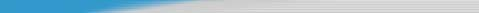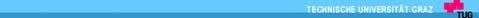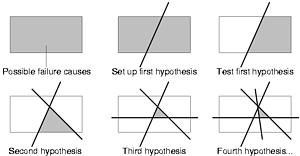 Have a large input that causes a failure (e.g., a core dump) but this input is to  Example: gcc takes input and crashes!
 Question: What is the smallest input
 Developed by Andreas Zeller et al.
Institute for Software Technology
Institute for Software Technology
double mult(double z[], int n) {
WHICH PART OF
BUG.C CAUSES
double mult(double z[], int n) {
CRASH?????
void copy(double to[], from[], int count) {
void copy(double to[], from[], int count) {
int main (.) { . return copy(y,x,SIZE);}
int main (.) { . return copy(y,x,SIZE);}
Institute for Software Technology
Institute for Software Technology
t(double z[],int n){int
 The resulting substring is substantially Institute for Software Technology
Institute for Software Technology Testing function that takes a program and tests whether it produces a failure. There are three different outcomes of such a testing function: test(x)
 The test produces the failure (FAIL, 8)  The test produces indeterminate results Delta Debugging
Institute for Software Technology
Institute for Software Technology
 Given:
 An input c‘ c is said to be the global  A testing function test(x) mapping an input minimum iff test(c‘) = 8 and there exists no c‘‘ with |c‘‘||c‘| where  An input c with test(c) = 8.
 Goal: Search for a smaller c‘ c so that
 An input c‘ c is said to be the local minimum iff test(c‘) = 8 and there exists no c‘‘c‘ where test(c‘‘) = 8.
Institute for Software Technology
Institute for Software Technology
Minimizing delta debugging algorithm
 Input: Let test and c be given such that
test(c)=8 and test()=9 hold.
 Output: A c‘ c such that test(c‘)=8
 An input c‘c is n-minimal iff test(c‘) = 8 and there exists no c‘‘c‘ with |c‘| - |c‘‘| ≤ n where test(c‘‘) = 8.
Institute for Software Technology
Institute for Software Technology ddmin2(c,n) =
ddmin2(,2) if ∃i∈{1,.,n}: test(∆ )=8
ddmin2(,max(n-1,2))
ddmin2(c,min(|c|,2n)) else if n<|c|
4. DONE otherwise
test(c)=8 and n|c| Institute for Software Technology
Institute for Software Technology
 ddmin returns a 1-minimal result.
 The complexity of ddmin is O(|c|2).
 In the best case the complexity of ddmin  test(x) = 8 iff “2“ ⊆ x c Institute for Software Technology
Institute for Software Technology
 test(x) = 8 iff “18“ ⊆ x c  Not only for inputs of type STRING  Extensions of ddmin can be used to Institute for Software Technology
Institute for Software Technology